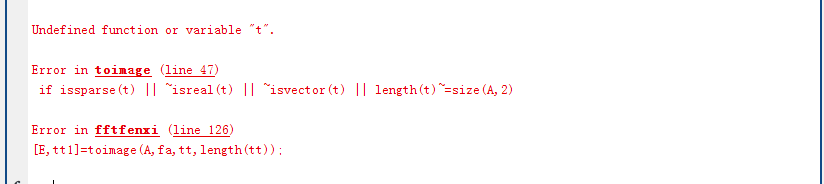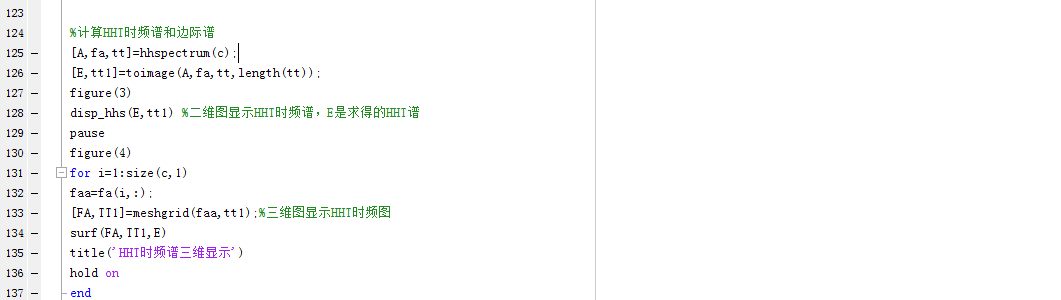function [im,tt,ff]=toimage(A,f,varargin)
DEFSPL=400;
error(nargchk(2,5,nargin));
switch nargin
case 2
t=1:size(A,2);
sply=DEFSPL;
splx=length(t);
case 3
if isscalar(varargin{1})
t=1:size(A,2);
splx=length(t);
sply=varargin{1};
else
t=varargin{1};
splx=length(t);
sply=DEFSPL;
end
case4
if isscalar(varargin{1})
t=1:size(A,2);
sply=varargin{1};
splx=varargin{2};
else
t=varargin{1};
sply=varargin{2};
splx=length(t);
end
case5
t=varargin{1};
splx=varargin{2};
sply=varargin{3};
end
if isvector(A)
A=A(:)';
f=f(:)';
end
if issparse(A)||~isreal(A)||length(size(A))>2
error('A argument must be a real matrix')
end
if issparse(f)||~isreal(f) || length(size(f))>2
error('f argument must be a real matrix')
end
if any(size(f)~=size(A))
error('A and f matrices must have the same size')
end
if issparse(t) || ~isreal(t) || ~isvector(t) || length(t)~=size(A,2)
error('t argument must be a vector and its length must be the number of columns in A and f inputs')
end
if~ isscalar(splx) || ~isreal(splx) ||splx~=floor(splx) ||splx<=0
error('splx argument must be a positive integer')
end
if~isscalar(sply) || ~isreal(sply) || sply ~=floor(sply) || sply<=0
error('splx argument must be a positive integer')
end
if any(diff(diff(t))) && splx~=length(t)
warning('toimage:nonuniformtimeinsants', 'when splx differs from length(t), the function only works for equally spaced time instants. You may consider reformating your data (using e.g. interpolation) before using toimage.')
end
f= min(f,0.5);
f=max(f,0);
indf= round(2*f*(sply-1)+1);
indt=repmat(round(linspace(1,length(t),splx)),size(A,1),1);
im= accumarray([indf(:),indt(:)],A(:),[sply,splx]);
indt=indt(1,:);
tt=t(indt);
ff=(0:sply-1)*0.5/sply+1/(4*sply);
end